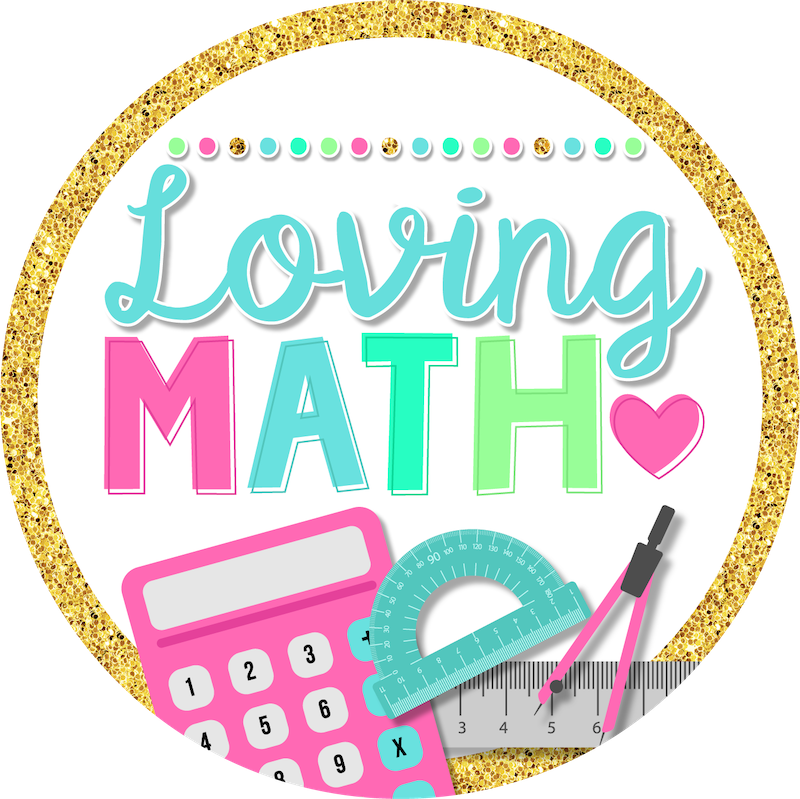# Powers of 10 Activities for 5th Grade

### Powers of 10 Activities for 5th Grade

Learn all about powers of 10 and how to identify the base number, exponent, and the meaning of “powers” in math.

5th grade students are introduced to powers of 10 early on in the school year. The Common Core Standards explains the learning targets for this skill as follows:

• Explain patterns in the number of zeros of the product when multiplying a number by powers of 10, and explain patterns in the placement of the decimal point when a decimal is multiplied or divided by a power of 10. Use whole-number exponents to denote powers of 10.

## WHAT IS A POWER OF 10?

According to mathematics, the power of ten is any of the integers powers of the number multiplied by 10. In simple words, it means that we multiply ten by itself a certain number of times (when the power is a positive integer). Moreover, in the definition of the number 1 is a power (the zeroth power) of ten.

## ACTIVITIES TO HELP STUDENTS MASTER THIS STANDARD

##### 1. Powers of 10 Google Slides

This Powers of Ten digital resource will engage your students and allow them to practice how to explain patterns in the number of zeros of the product when multiplying a number by powers of 10, and explain patterns in the placement of the decimal point when a decimal is multiplied or divided by a power of 10. They will also use whole-number exponents to denote powers of 10.

These centers are a perfect solution for those students that are doing remote learning. They can also be used in class using any device with an internet connection.

Includes:

• 14 interactive slides
• Instructions on how to assign

##### 2. Powers of 10 Digital Quiz

This fifth grade digital Powers of Ten quiz is a great tool to assess powers of 10 mastery in fifth grade. Find out what your students know and collect data to group your students.

This test assesses fifth grade place value skills according to the Common Core Standards. (5.NBT.2)

Includes:

• 10 multiple choice questions

What’s assessed:

Numbers & Operations of Base Ten

• Explain patterns in the number of zeros of the product when multiplying a number by powers of 10
• Use whole-number exponents to denote powers of 10.
##### 3. Powers of 10 Song

This song targets TEKS and Common Core learning standards for 5th grade. Look into the relevant standards here, or dig deeper into Powers of Ten here.##### 4. Powers of Ten Bingo

This game focuses on multiplying and dividing decimals and whole numbers by powers of 10 (exponents) with this BINGO game. Question cards include multiplying and dividing decimals and whole numbers by powers of 10. This is cooperative game where students can play a game of BINGO in a small group station or as whole group.

Hope you find these activities helpful!